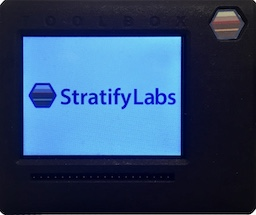# Controlling a Solenoid###### Introducing the Stratify Toolbox

Are you a printf()-er or a code-stepper? The Stratify Toolbox is a printf()-ing awesome debug tool!Many products use solenoids to open and close valves. A microcontroller can control solenoids programmatically using a GPIO pin and a simple circuit.

## Circuit

A solenoid can be enabled using a simple transistor circuit and a microcontroller. The circuit above shows a solenoid, MOSFET transistor, diode, and a microcontroller GPIO signal. To activate the solenoid, the microcontroller GPIO signal goes high causing the MOSFET to turn on. When on, the MOSFET allows current to flow from the power supply through the solenoid and to ground. The voltage rating for the solenoid dictates the voltage of the power supply (it is OK if the power supply voltage is different from the microcontroller voltage). The MOSFET should be selected to handle sufficient current to activate the solenoid as specified in the solenoid’s datasheet.

Because the solenoid is an inductive device, the diode is added to protect the MOSFET. When the solenoid turns off, the current through the solenoid rapidly drops from the nominal value to zero. Since the voltage drop across the solenoid is proportional to the change in current (see equation below), the current drop creates a voltage spike which can damage the MOSFET. The diode prevents the voltage spike from reaching damaging levels by directing the current back to the power supply.

$V_L = L \cdot \frac{di}{dt}$

## Firmware Considerations

Controlling the solenoid in firmware is a straightforward operation with one exception. As mentioned, driving the GPIO high will turn the solenoid on, while driving it low will turnit off. However, there is a small amount of time while themicrocontroller starts up where the solenoid may open unexpectedly. For example, if the default GPIO configuration is to have an internal pull-up activated, the solenoid will be activated while themicrocontroller is starting up. To avoid this behavior, an external pull-down resistor can be added to the GPIO line (shown below) to ensure the solenoid stays off during microcontroller initialization.## Conclusion

Controlling solenoids with microcontrollers is simple. Remember to ensure the MOSFET has sufficient current capability to activate the solenoid, include the protection diode to prevent the solenoid from damaging the MOSFET, and include a pull-down resistor on the GPIO line if the microcontroller doesn’t drive the line low at startup.

X